# Convolutional Neural Networks dimension

### convolution operation: share the convolution core

output size is: $$O = (n-f+1) * (n-f+1)$$ where input size is $n\times n$, convolution core size is $f\times f$.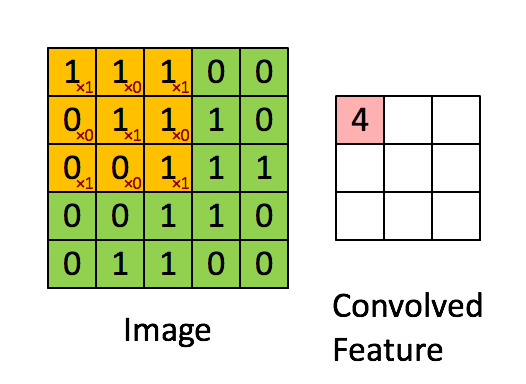• channels: look following picture, the input is $6\times 6\times 3$ RGB picture, and we use $3\times 3\times 3$ convolution core, the last 3 is the channel number of convolution core, which is same as the input picture channel number. During convolution, we multiply the input and add it together , so the output size is $4\times 4\times 1$.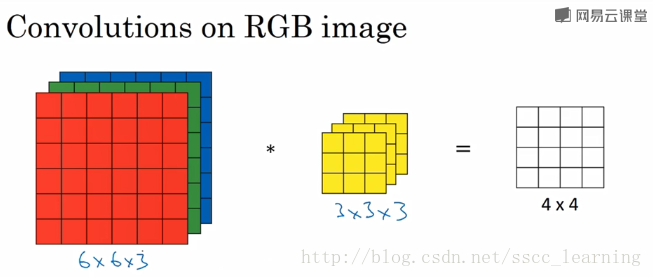and we often not only use only one convolution core, we use multi-cores to get multi-features form input. The following image shows the situation that we use two cores, so the output size is $4\times 4\times 2$. Then the input channel of following convolution layer is 2.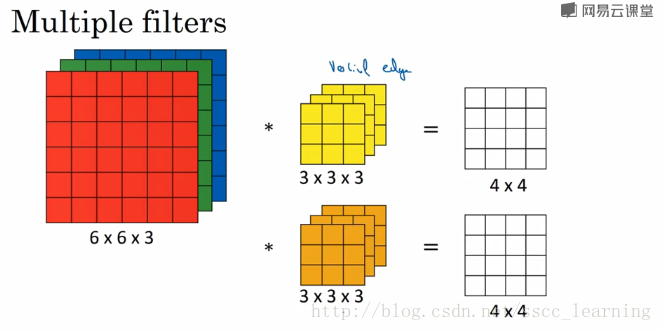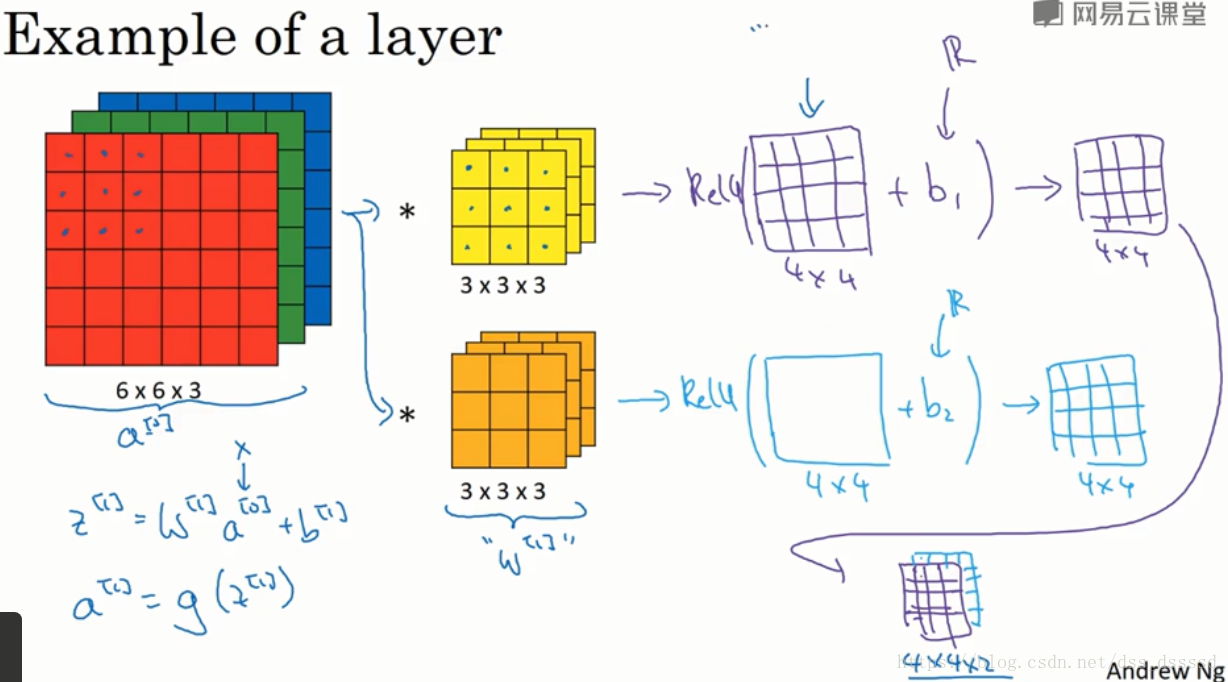output size: $$O =(n-f+1) * (n-f+1) * C_o$$ where $C_o$ is the number of convolution cores(output channel).

• padding: add data to the border of input, often to make sure the output size is same as input.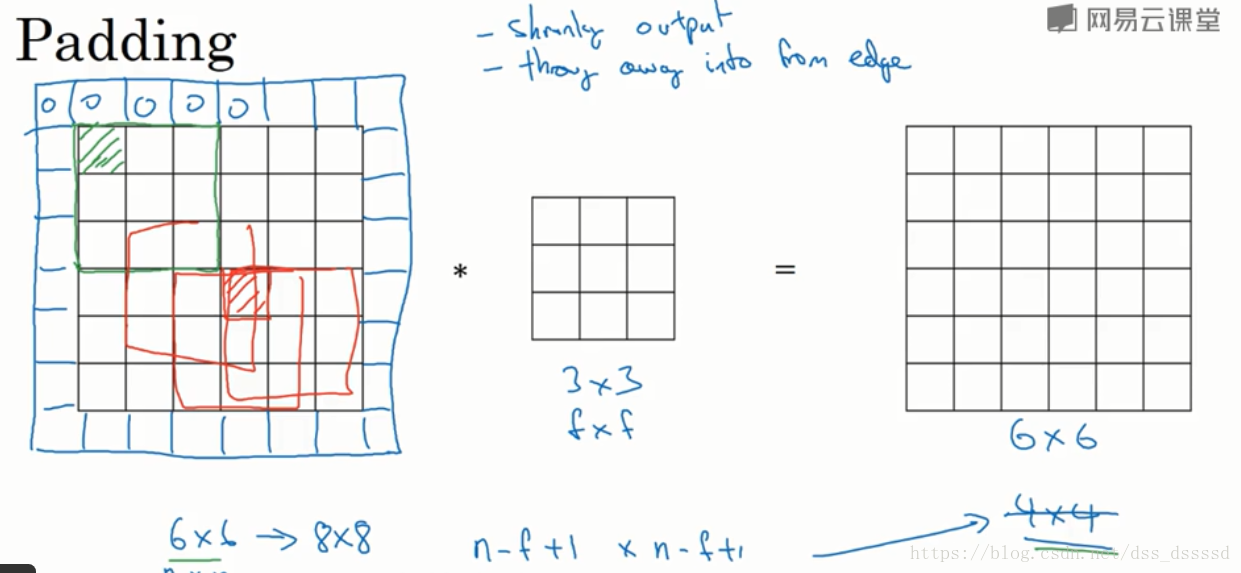output size: $$O =(n+2p-f+1) * (n+2p-f+1) * C_o$$ where $p$ is padding length of one side. e.g. the last picture $p = 1$.

if you want to make sure output size is same as input, set $p = (f-1)/2$, since at this moment, $n-f+2p+1 = n$.

• strides: stripe is the moving step length of convolution core.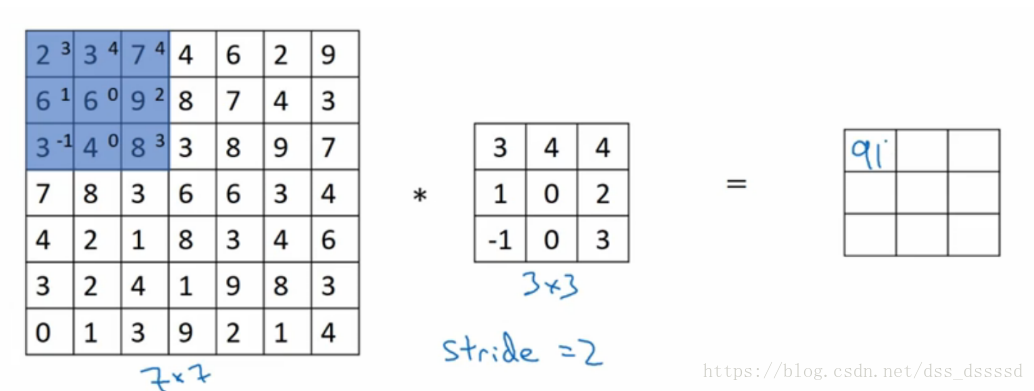output size: $$O =(\frac{n+2p-f}{s}+1) * (\frac{n+2p-f}{s}+1) * C_o$$ where $s$ is stride length.

### demo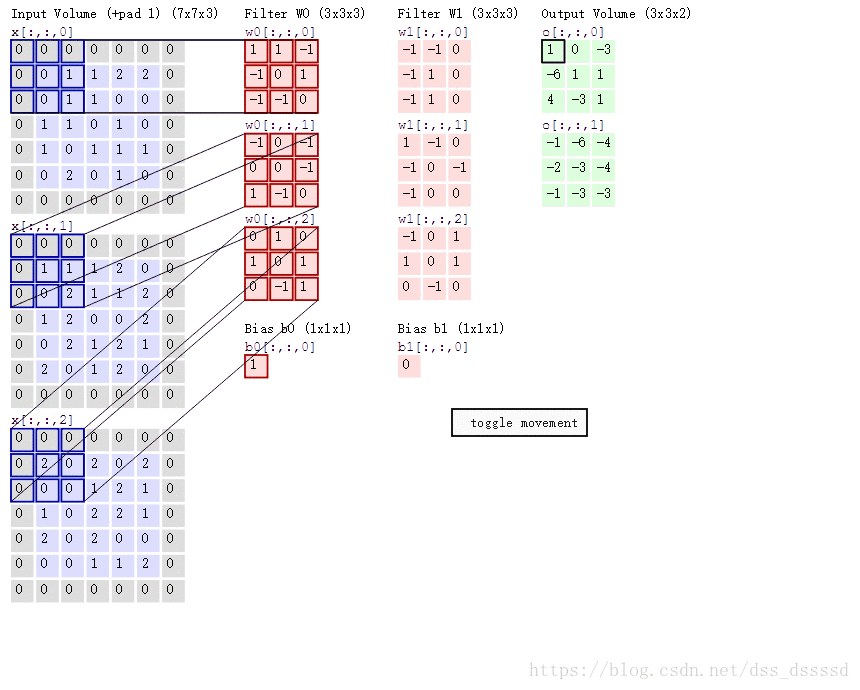So lets get the demo’s output size:

\begin{equation} \begin{aligned} p=1\\ s=2\\ S_{input} &= 7*7*3\\ S_{core} &= 3*3*3\\ S_{out} &= (\frac{n+2p-f}{s}+1) * (\frac{n+2p-f}{s}+1) * C_o \\ &= (\frac{7+2-3}{2}+1) * (\frac{7+2-3}{2}+1) * 2 \\&= 4*4*2 \end{aligned} \end{equation}

Tags:

Updated: